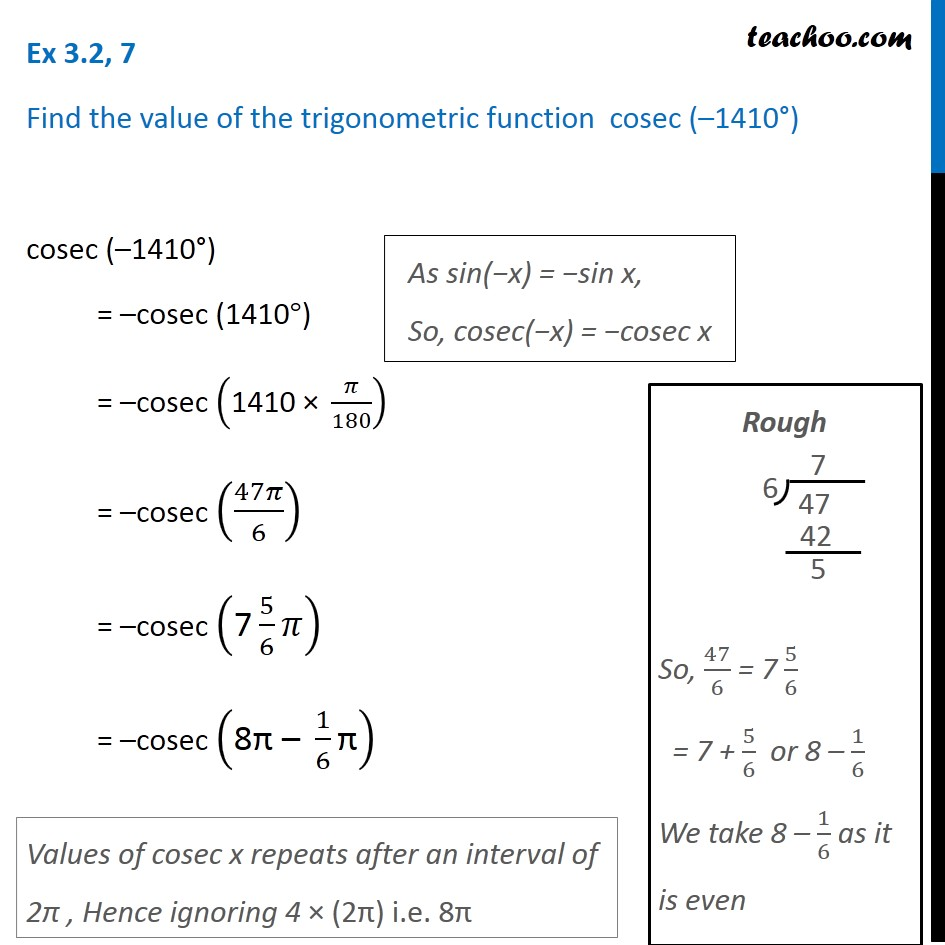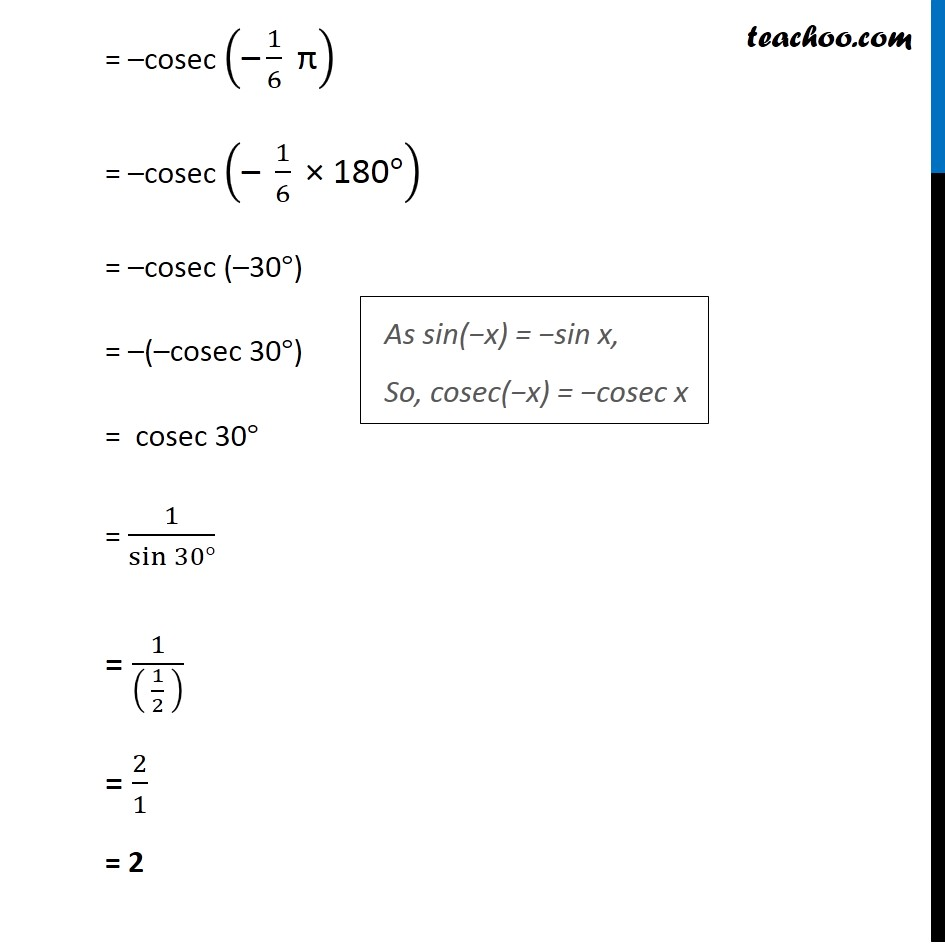Learn All Concepts of Chapter 2 Class 11 Relations and Function - FREE. Check - Trigonometry Class 11 - All Concepts1. Chapter 3 Class 11 Trigonometric Functions
2. Concept wise
3. Finding Value of trignometric functions, given angle

Transcript

Ex 3.2, 7 Find the value of the trigonometric function cosec (–1410°) cosec (–1410°) = –cosec (1410°) = –cosec ("1410 × " 𝜋/180) = –cosec (47𝜋/6) = –cosec ("7" 5/6 𝜋) = –cosec ("8π – " 1/6 "π" ) As sin(−x) = −sin x, So, cosec(−x) = −cosec x Rough So, 47/6 = 7 5/6 = 7 + 5/6 or 8 – 1/6 We take 8 – 1/6 as it is even Values of cosec x repeats after an interval of 2π , Hence ignoring 4 × (2π) i.e. 8π = –cosec ("–" 1/6 " π" ) = –cosec ("– " 1/6 " × 180" °) = –cosec (–30°) = –(–cosec 30°) = cosec 30° = 1/sin⁡〖30°〗 = 1/(( 1/2 ) ) = 2/1 = 2 As sin(−x) = −sin x, So, cosec(−x) = −cosec x

Finding Value of trignometric functions, given angle

About the AuthorDavneet Singh
Davneet Singh is a graduate from Indian Institute of Technology, Kanpur. He has been teaching from the past 9 years. He provides courses for Maths and Science at Teachoo.